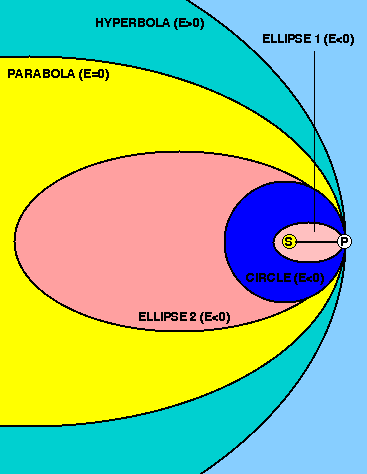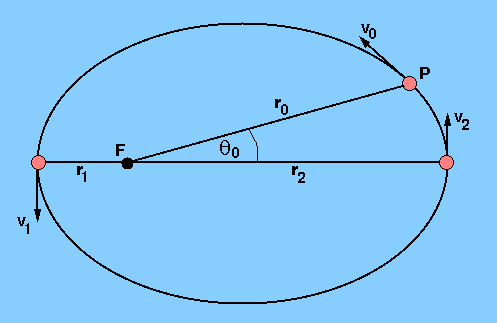It is sometimes more useful to think of orbital motion in terms of the energy of a celestial body rather than in terms of the forces that are acting on it. In particular, we will concern ourselves with kinetic energy - which represents the energy associated with a body due to its motion - and (gravitational) potential energy - which represents the energy possessed by a system by virtue of the relative positions of its component parts. If we imagine a satellite of mass m a distance r from the centre of its planet of mass M (M>>m) moving at a speed v. Then using Newton's law of gravitation and equating it to the centripetal force, we find:

GMm/r2 = mv2/r.

The kinetic energy of the satellite, Ek, is given by

Ek = ½mv2 = GMm/2r,

which shows that Ek is always positive. The gravitational potential energy, Ep is given by

Ep = - GMm/r.

Ep is always negative because gravitational forces are attractive and Ep=0 when the satellite is at an infinite distance away from the planet.

The total energy of the satellite, E, is then given by

E = Ek + Ep = - GMm/2r.

The total energy of the satellite is hence negative, which means that the satellite will never be able to escape from the gravitational pull of the planet.

The law of conservation of energy tells us that, in the absence of forces other than gravitational forces, the sum of the kinetic energies and mutual potential energy of the bodies in an isolated system remains constant, i.e.

E = constant.

This means that as the satellite moves in its orbit around the planet, its kinetic and potential energy may be interchanged, but the total energy of its orbit remains constant. At periapsis, when the satellite is at its closest to the planet and hence moving at its fastest, the kinetic energy component of the total energy is at its greatest and the gravitational potential energy is at its minimum (i.e. its most negative value). As the satellite climbs out of the gravitational potential well of the planet towards apoapsis, gaining potential energy as it does so, it is slowing down and hence losing kinetic energy.

We are now in a position to consider the variety of orbits that correspond to different values of the total energy. Let us suppose that a particle is launched from P in a direction at right angles to the line SP from the Sun at S, as shown in Figure 39.

 figure 39: A family of orbits around the Sun of different total energy.If the launching speed is close to zero, the energy of the particle is significantly negative and the particle follows an almost straight line towards S; strictly, it would be an elliptical path with an extremely small width and a major axis only very slightly longer than SP.

At a slightly higher launching speed, the energy of the particle would be less negative and the orbit would resemble an ellipse. The launching point P would be the aphelion. At some higher, but still negative, value of the energy, the orbit would be a perfect circle with S at the centre. A still further increase of energy would lead to an elliptical orbit once again, but now P is the perihelion. Ultimately, a situation is reached where the total energy is precisely zero. The orbit in this case is a parabola. The particle moves further and further from the Sun, approaching infinite distance with vanishingly small speed. Any further increase in launching speed gives the particle a positive energy and produces a trajectory that is a hyperbola - the particle now approaches infinity with a significant speed.

Hence it can be seen that orbits with a negative total energy are either circular or elliptical in shape. These are known as bound orbits. An orbiting body in a bound orbit cannot escape unless energy is supplied to make the total energy positive. When the total energy of an orbit is positive, it is known as an unbound orbit, and it is parabolic or hyperbolic in shape. A particle in a parabolic or hyperbolic orbit will never return to the Sun.

It can be seen from Figure 39 that, near P, it is quite difficult to tell whether the orbit of a particle is a circle, an ellipse, a parabola or a hyperbola. This can be a problem when astronomers attempt to analyse the orbits of comets and asteroids; the bound orbit of a comet with a total energy only slightly less than zero is almost indistinguishable from that of a hyperbolic orbit of small positive energy if the comet is visible only when it penetrates the inner regions of the solar system.

We can now put together all that we have learnt about celestial mechanics to calculate an orbit of a particle given some initial conditions. Suppose that a particle of mass m is launched with a velocity v0 from a point P, at a distance r0 from the centre of force F (of mass M), as shown in Figure 40. How do we deduce the size, shape, and orientation of the subsequent orbit?

 figure 40: Calculating an orbit from initial conditions.The first thing to do is to test whether the total energy is positive or negative (or perhaps, by chance, zero). Only if it is negative will we have a bounded orbit, and we shall limit our attention here to such cases, i.e. to closed elliptical (or circular) orbits. From the values of v0 and r0 we know the total energy of the particle:

E = ½mv20 - GMm/r0 = - GMm/2r0.

But, recalling from our study of the ellipse that the semi-major axis, a, of an elliptical orbit is equal to the mean distance of the particle from the centre of force, and given that the total energy of the orbit is conserved, we can write

E = - GMm/2a.

Thus we know the distance a.

Next, we can use the fact that the directions of v and r are orthogonal at periapsis (r1 and v1) and apoapsis (r2 and v2) to write the scalar magnitude of the angular momentum, L, which is conserved, as

L = mv1r1 = mv2r2

or

1/r1 = mv1/L,      1/r2 = mv2/L.

We can insert the value of 1/r as defined by either of these equations in the expression for the total energy at apoapsis or periapsis:

½mv21 - GMm2v1/L = - GMm/2a.

This quadratic, when solved, has as its roots the value of v1 in terms of the other (known) quantities in the equation. v2 can be found in a similar manner and it is then a simple matter to deduce r1 and r2 using the angular momentum equation given above. This then fixes the eccentricity of the ellipse, through the relations

r1 = a(1-e),      r2 = a(1+e).

Finally, the orientation of the major axis, relative to which the initial position vector makes an angle0, is determined through the polar equation of an ellipse derived earlier. r0 = a (1 - e2) / (1 + e cos).

Thus the orbit is completely specified.

Up to now, we have been concerned with the orbits of two bodies acting under the influence of their mutual gravitational attraction. When Newton formulated and solved this two-body problem and successfully applied it to problems involving the orbits of the planets around the Sun and the Moon around the Earth, he owed his success to two fortunate circumstances. First, the planetary masses are all small compared to the Sun's mass, just as the masses of the satellites are small compared to the masses of their planets. Hence the mutual gravitational attractions of the planets are small in comparison to the Sun's force of attraction on each planet. Second, the diameters of the planets are small compared with their distances from each other and from the Sun. Newton realized, of course, that for accurate work in celestial mechanics, the attraction of the other planets and their shapes and internal constitutions had to be taken into account. Solutions to this many-body problem have occupied the minds of the most eminent astronomers and mathematicians for the last three centuries. This is a vast and complicated subject and lies well outside the scope of the present course. For further reading, see Chapter 14 of Roy and Clarke.